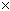From: DeAnna Question: A 102" board is cut into 2 pieces. One piece is two times the length of the other. Find the lengths of the two pieces. Hi DeAnna. The board is cut into two pieces: a short length and a long length. So we'll start by using the letter S to represent the short length and the letter L to represent the long length. Let's write down what you know from the question: The two boards together are 102", so S + L = 102. The long piece is twice the length of the short piece: L = 2S Now we take the first equation and replace L with what L is equal to (the second equation): S + L = 102 becomes S + ( 2S ) = 102. Now can you solve the rest? Sue